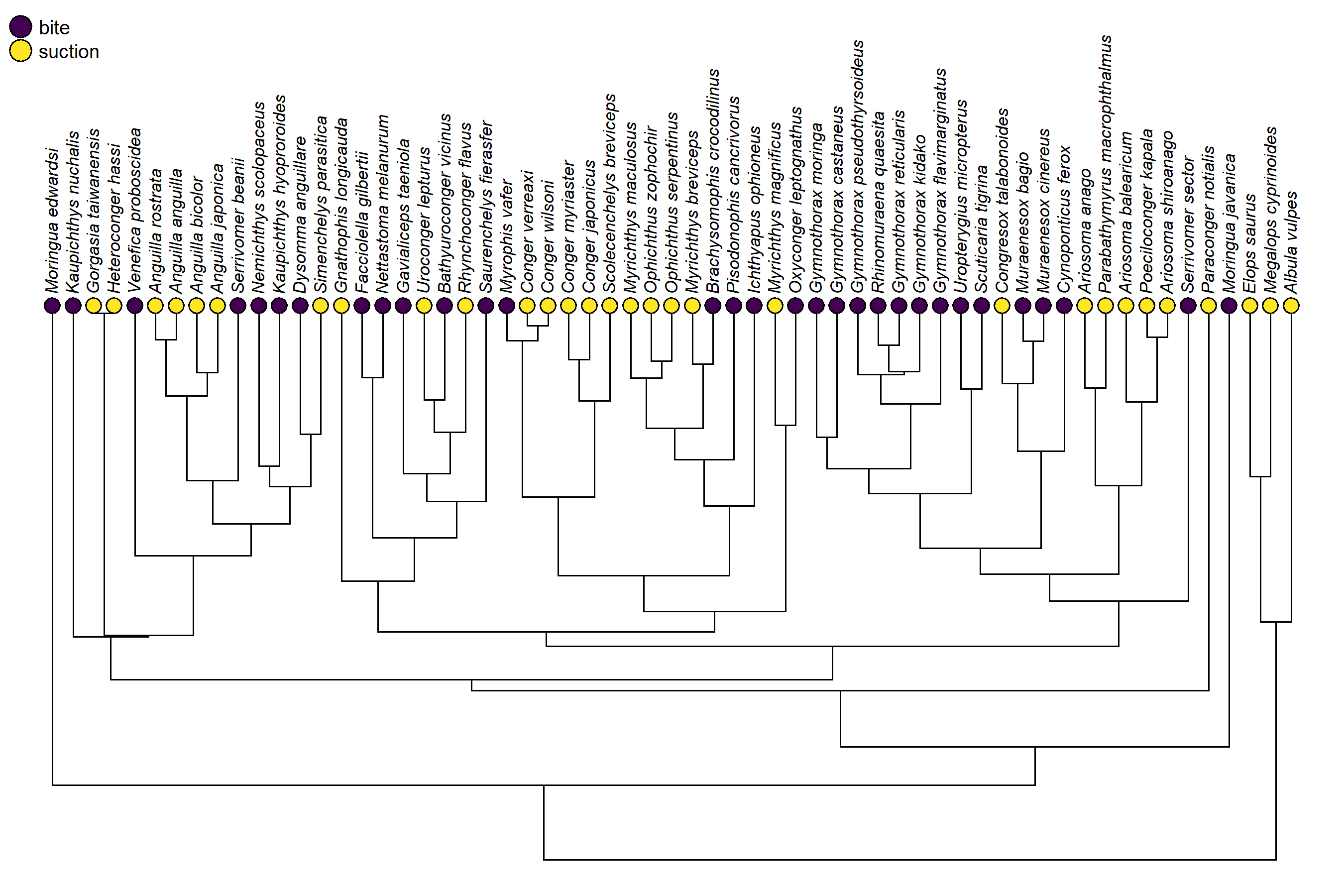## Tuesday, March 21, 2023

### Simple demonstration of Felsenstein's pruning algorithm in R to compute the likelihood of a discrete character on the tree

All software that fits an Mk model to discrete character data on the tree uses a method called the pruning algorithm that was originally described by Felsenstein (1973). The pruning method is an efficient dynamic programming algorithm that can be used to compute the probability of a set of states (e.g., a nucleotide site pattern) at the tips of the tree given a transition rate (q) or set of transition rates (Q) among states.

In phytools functions that use the pruning algorithm (such as `fitMk`), I use code that was adapted (with attribution) from an implementation of the pruning algorithm in `ape::ace`; however, it’s not that difficult to code.

Since the pruning algorithm requires a post-order traversal of the tree, and its key that we not visit any parent node before we’ve visited all of its daughters, we use `ape::reorder.phylo` to rearrange the `edge` matrix of our `"phylo"` object in post-order fashion. The rest is quite easy.

Here’s a simple demonstration. (Please excuse any problematic bookkeeping. This chunk is for learning purposes only!)

``````pruning<-function(q,tree,x,model=NULL,...){
pw<-reorder(tree,"postorder")
k<-length(levels(x))
if(is.null(model)){
model<-matrix(1,k,k)
diag(model)<-0
}
if(hasArg(pi)) pi<-list(...)\$pi
else pi<-rep(1/k,k)
Q<-matrix(0,k,k)
Q[]<-c(0,q)[model+1]
diag(Q)<--rowSums(Q)
L<-rbind(to.matrix(x[pw\$tip.label],levels(x)),
matrix(0,tree\$Nnode,k,dimnames=
list(1:tree\$Nnode+Ntip(tree))))
nn<-unique(pw\$edge[,1])
for(i in 1:length(nn)){
ee<-which(pw\$edge[,1]==nn[i])
PP<-matrix(NA,length(ee),k)
for(j in 1:length(ee)){
P<-expm(Q*pw\$edge.length[ee[j]])
PP[j,]<-P%*%L[pw\$edge[ee[j],2],]
}
L[nn[i],]<-apply(PP,2,prod)
}
prob<-log(sum(pi*L[nn[i],]))
prob
}
``````

Note that this function is not designed for efficiency (e.g., in maximizing the likelihood), since it includes a lot of computations (including re-ordering the tree) that could’ve been done just once, instead of in each iteration of optimization!

Let’s test it out, as follows.

``````library(phytools)
data(eel.data)
data(eel.tree)
``````

Since our function `pruning` computes the probability of a set of character values at the tips for a given set of transition rates, if we maximize it over possible values for those rates, we should find the MLE.

``````head(eel.data)
``````
``````##                   feed_mode Max_TL_cm
## Albula_vulpes       suction       104
## Anguilla_anguilla   suction        50
## Anguilla_bicolor    suction       120
## Anguilla_japonica   suction       150
## Anguilla_rostrata   suction       152
## Ariosoma_anago      suction        60
``````
``````feeding_mode<-setNames(eel.data\$feed_mode,rownames(eel.data))
plotTree(eel.tree,lwd=1,ftype="i",direction="upwards",offset=1,
fsize=0.7)
tiplabels(pie=to.matrix(feeding_mode[eel.tree\$tip.label],
levels(feeding_mode)),cex=0.3,
piecol=viridisLite::viridis(n=2))
legend("topleft",levels(feeding_mode),pch=21,pt.cex=2,
pt.bg=viridisLite::viridis(n=2),bty="n",cex=0.8)
``````Let’s create a model design, and then fit our model using a call of the R base optimizer, `optim`.

``````model<-matrix(c(0,1,2,0),2,2,byrow=TRUE)
fitted<-optim(c(1,1),pruning,tree=eel.tree,x=feeding_mode,
model=model,method="L-BFGS-B",lower=1e-12,
control=list(fnscale=-1))
fitted
``````
``````## \$par
##  0.01562150 0.01749247
##
## \$value
##  -37.00365
##
## \$counts
##       46       46
##
## \$convergence
##  0
##
## \$message
##  "CONVERGENCE: REL_REDUCTION_OF_F <= FACTR*EPSMCH"
``````

Finally, let’s compare this to what we might obtain if we fit the same model using `phytools::fitMk`.

``````fitMk(eel.tree,feeding_mode,model="ARD")
``````
``````## Object of class "fitMk".
##
## Fitted (or set) value of Q:
##              bite   suction
## bite    -0.015609  0.015609
## suction  0.017491 -0.017491
##
## Fitted (or set) value of pi:
##    bite suction
##     0.5     0.5
## due to treating the root prior as (a) flat.
##
## Log-likelihood: -37.00365
##
## Optimization method used was "nlminb"
``````

Neat.